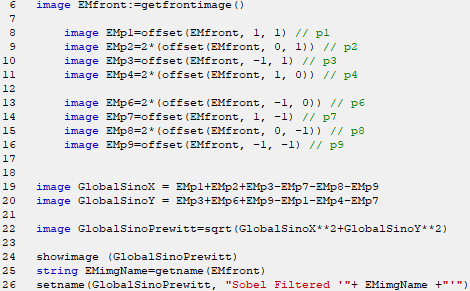Sobel Edge Filter (Operator)
- Practical Electron Microscopy and Database -
- An Online Book -
Microanalysis | EM Book                                                                                   http://www.globalsino.com/EM/

=================================================================================

Similar to Prewitt Edge Filter, the Sobel Edge Filter, sometimes called the Sobel–Feldman operator, is also an operator used in image processing, particularly within edge detection algorithms (approximations of the derivatives). It computes a discrete approximation of the gradient of the image intensity function, and consists of a horizontal filter and a verticle filter and is used to detect edges of images. Both Sobel and Prewitt Filters are used extensively for detecting edges in image processing.

The Sobel edge filter calculates the gradient of the image intensity at each point. Therefore, the resulting profile shows how “abruptly” or “smoothly” the image changes at that point, and thus it represents the edge. In Sobel Edge Filters, both horizontal and verticle filters are applied to the same image and then summed to form the final image as a final filter. The two filters are basic convolution filters in the form in Figure 1005a.

 1 2 1 0 0 0 -1 -2 -1
 -1 0 1 -2 0 2 -1 0 1
(a)
(b)
 Figure 1005a. Sobel edge filter: (a) horizontal filter (y-direction) and (b) verticle filter (x-direction).

Figure 1005b shows an filter example. The filter is centered at pixel p5 here. Based on Figures 1005a and 1005b, the value of the resulting new pixel p5 is then given by,
[x][y] = (p1+p2+p3-p7-p8-p9)+(p3+p6+p9-p1-p4-p7) ---------------- [1005a]
This filter identifies the areas of high slope in the input image through the calculation of slopes in the x and y directions. The value of p5 is finally scaled to the 0-255 range.

 p1 p2 p3 p4 p5 p6 p7 p8 p9
 [x-1][y-1] [x][y-1] [x+1][y-1] [x-1][y] [x][y] [x+1][y] [x-1][y+1] [x][y+1] [x+1][y+1]
(a)
(b)
 Figure 1005b. Sobel edge filter: (a) filter with a 3×3 window, and (b) coordinates of the filter with the 3×3 window.

The actual forumla uses the horizontal and verticle components to obtain the the final form,
[x][y] = sqrt((X*X)+(Y*Y)) ---------------- [1005b]
where,
X = (p1+p2+p3-p7-p8-p9)  ---------------- [1005c]
Y = (p3+p6+p9-p1-p4-p7) ---------------- [1005d]

Therefore, the Sobel edge filter estimates the maximum gradient of the edges, and can be used to find edge directions. In DM, the Sobel edge filter can be programmed with scripts as shown in Table 1005.

Table 1005. Sobel edge filter in DM.

 Script Note◆ Script code in .s format ◆ The signs in the script are reversed from the ones in Figure 1005b since the image shifts caused by offset() are opposite to the signs in Equations 1005c and 1005d. Example  Original image  (fuel cell nanoparticles on carbon film)Processed image with the Sobel edge filter above=================================================================================